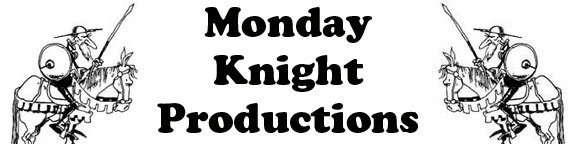Sea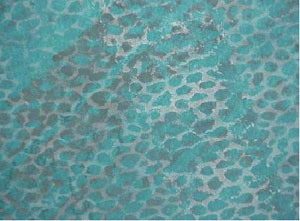Plain - \$40.00 Each = Star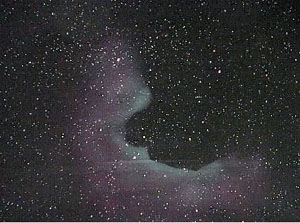Plain - \$40.00 Each = Sky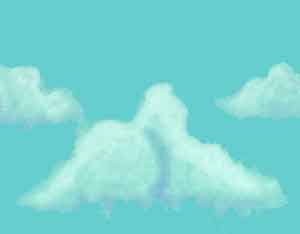Plain - \$40.00 Each =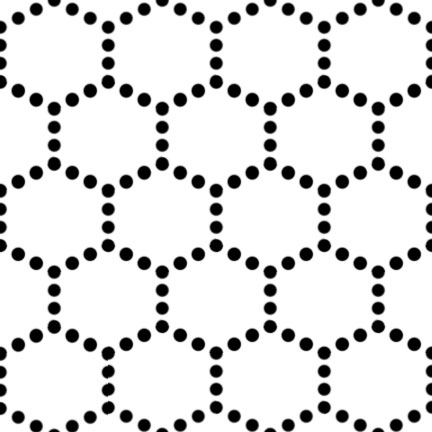1 1/2" Hexed - \$47.50 Each =1 1/2" Hexed - \$47.50 Each =1 1/2" Hexed - \$47.50 Each =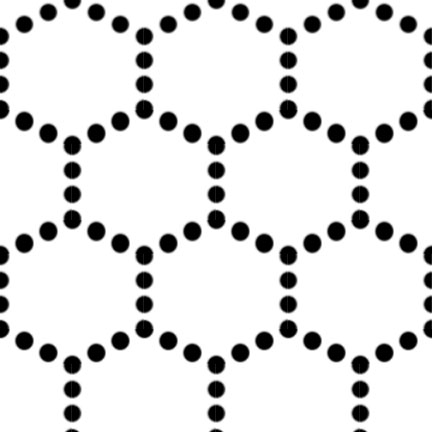2" Hexed - \$47.50 Each =2" Hexed - \$47.50 Each =2" Hexed - \$47.50 Each =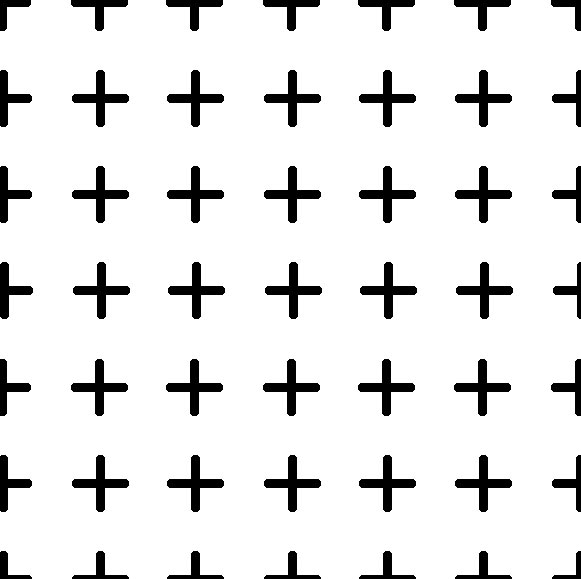1" Grid- \$47.50 Each =1" Grid- \$47.50 Each =1" Grid- \$47.50 Each =